# Math Practice Topic: Triangle Angles 2

 Description: This topic covers the idea of finding missing angles using properties of supplementary angles Adaptive Learning Progression: The way in which you must solve for x becomes more difficult. Start using MathScore for free

## Sample Levels (out of 4)

Find the value of 'x'. Diagrams are not always to scale.

 1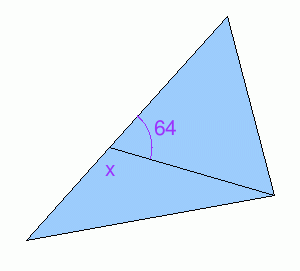x = 2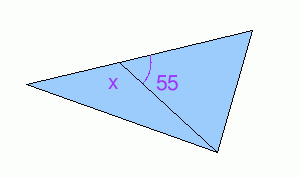x =

Find the value of 'x'. Diagrams are not always to scale.

 1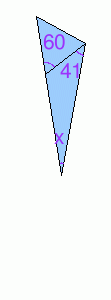x = 2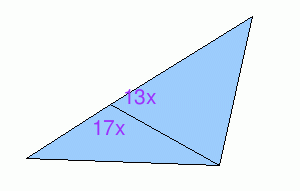x = 3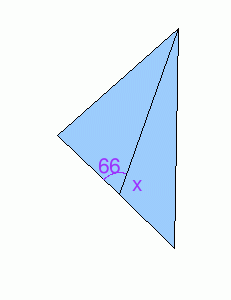x =